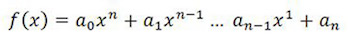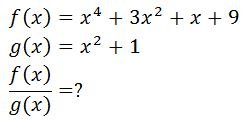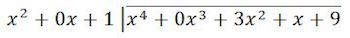# Dividing Functions: Examples & Overview

Lesson Transcript
Instructor: Yuanxin (Amy) Yang Alcocer

Amy has a master's degree in secondary education and has taught math at a public charter high school.

In this lesson, learn how to use long division to divide functions. Learn how the process is similar to using long division for numbers. Also learn what kinds of functions you can divide.

## Dividing Polynomials

In math, when you read or hear about dividing functions, they are usually talking about dividing polynomials. Recall that polynomials are functions of the following form:When you divide two such functions together, you get what is called a rational expression. A rational expression is the division of two polynomials. If they divide evenly, your answer will become a polynomial.

I will show you how long division works for dividing polynomials. You can't divide a polynomial with another polynomial whose degree or exponent is larger. Long division of functions uses a very similar process to long division of numbers, as we will see. It uses a circular pattern of comparing, multiplying, subtracting, and carrying down. Let's see how it works by dividing function f by function g.Remember, our function includes all the terms and not just the first term. So function f(x) is not just x^4, but x^4+3x^2+x+9. Likewise our function g(x) is not just x^2, but x^2+1.

An error occurred trying to load this video.

Try refreshing the page, or contact customer support.

Coming up next: End Behavior in Math: Definition & Rules

### You're on a roll. Keep up the good work!

Replay
Your next lesson will play in 10 seconds
• 0:00 Dividing Polynomials
• 1:26 Setting Up the Problem
• 3:13 The Long Division Process
• 5:46 Lesson Summary
Save Save

Want to watch this again later?

Timeline
Autoplay
Autoplay
Speed Speed

## Setting Up the Problem

First, we need to set up the problem for long division. Remember from regular long division that the top number goes inside the division bracket. In our case, the top number is our function f(x). When we set up the functions though, we need to add in our zero values. For numbers, when we have a zero value, we have a zero in its place.

For example, 101 has a zero in the tens place because it doesn't have any tens. Look at our g function. It doesn't have an x value, so we need to add in a zero for that zero value. For our f function, it doesn't have an x^3 value, so we have to add in a zero for that as well. Our long division properly set up looks like this:Look at the zero values now. Do you see how we put in the zeros where our polynomial didn't have anything there? For the x^2+1 function, did you notice that we didn't have an x value? Because we didn't, we need to put in a 0x as a placeholder just like we do with numbers. For the number one hundred and one we write it out as 101 and not 11. We put in the zero as a placeholder for the tens place even though the number 101 does not have a tens value. It's a similar thing when it comes to dividing polynomials.

Before we begin the long division process, I want to point out to you one difference between dividing functions and dividing numbers. When dividing numbers, you generally look at and compare all the digits in the numbers. This is not so with functions. It's a bit easier with functions actually as you are only concerned about the first terms at every step. Even though we concern ourselves with only the first terms at every step, as we go along, we will have taken care of all the terms by the time we are done.

To unlock this lesson you must be a Study.com Member.

### Register to view this lesson

Are you a student or a teacher?